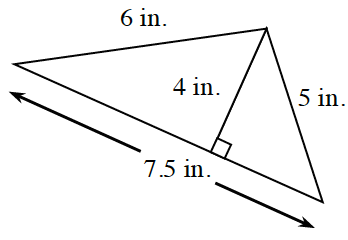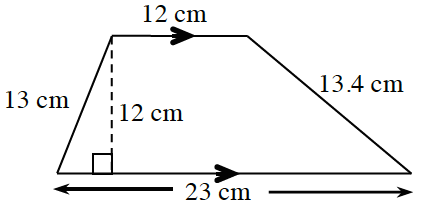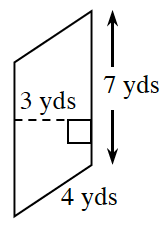### Home > CCAA > Chapter 1 CC2: Unit 1 > Lesson CC2: 1.1.2 > Problem1-11

1-11.

Find the perimeter and area of each figure below. Review the Math Notes box in this lesson for help. Be sure to include the correct units in your answers.

1.1.1.• To find the perimeter, add together all of the lengths of the outside edges.

The area of a triangle is:

$\frac {1}{2} \times \text{ base}\times \text{ height}$

Perimeter: $18.5$ inches
Area: $15$ square inches

• Look back at the Math Notes boxes.

Can you find the top and bottom "bases" of the trapezoid?
Can you find the height of the trapezoid?

$\text{Area of a trapezoid}=$
$\frac{(\text{top}+\text{bottom})}{2}\times\text{height}$

$\text{Perimeter} = \text{Sum of all side lengths}$

Perimeter: $61.4$ cm
Area: $210$ square cm

• Look back at the Math Notes.
What is the base?
What is the height?

$\text{Area of a parallelogram}=\text{base}\times \text{height}$

Perimeter: $22$ yards
Area: $21$ square yards